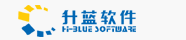# 如何處理資料庫中的Null

March 25,2004

Empty

Dim varTest
Response.Write TypeName(varTest)

Dim varTest
Response.Write CLng(varTest)
Response.Write CStr(varTest)

varTest = Empty

If varTest = Empty Then
Response.Write "The variable is empty."
End If

If IsEmpty(varTest) Then
Response.Write "The variable is empty."
End If

Null

Null 這個資料子類型 和 Empty 很類似, 但不同點在於 Empty 代表一個變數尚未被初始化, 也就是還沒有被賦予任何的值, 而一個變數為 Null 只有在你指定它為 Null 之後。最常遇到 Null 的機會應該是在處理資料庫的時候, 當一個欄位沒有資料時, 便是 Null

varTest = Null

Dim varTest
varTest = Null
If varTest = Null Then
Response.Write "The variable has a Null value."
End If

Dim varTest
varTest = Null
If IsNull(varTest) Then
Response.Write "The variable has a Null value."
End If

Dim varTest
varTest = Null
varTest = CLng(varTest)

Dim varTest
Dim lngTest
varTest = Null
lngTest = 2 + varTest
Response.Write TypeName(lngTest)

lngQty = oRs("Quantuty")
If IsNull(lngQty) Then
lngQty = 0
End If